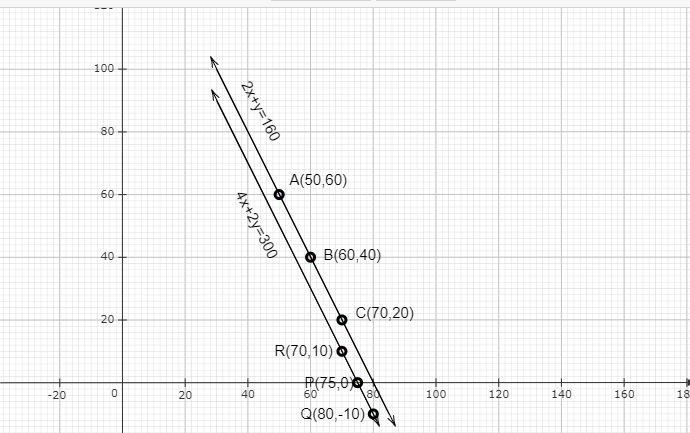# The cost of 2 kg of apples and 1 kg of grapes on a day was found to be Rs 160. After a month, the cost of 4 kg of apples and 2 kg of grapes is Rs 300. Represent the situation algebraically and geometrically.

Given:

The cost of 2 kg of apples and 1 kg of grapes on a day was found to be Rs 160. After a month, the cost of 4 kg of apples and 2 kg of grapes is Rs 300.

To do:

We have to represent the above situation algebraically and graphically.

Solution:

Let $x$ be the cost of 1 kg of apples and $y$ be the cost of 1 kg of grapes.

According to the question,

The cost of 2 kg of apples and 1 kg of grapes initially is given by,

$2x+y=160$

$\Rightarrow 2x+y-160= 0$.....(i)

$y=160-2x$

The cost of 4 kg of apples and 2 kg of grapes after a month is given by,

$4x+2y=300$

$\Rightarrow 4x+2y-300=0$......(ii)

$\Rightarrow 2y=300-4x$

$\Rightarrow y=\frac{300-4x}{2}$

To represent the above equations graphically we need at least two solutions for each of the equations.

For equation (i),

If $x=50$ then $y=160-2(50)=160-100=60$

If $x=60$ then $y=160-2(60)=160-120=40$

If $x=70$ then $y=160-2(70)=160-140=20$

 $x$ $50$ $60$ $70$ $y=160-2x$ $60$ $40$ $20$

For equation (ii),

If $x=70$ then $y=\frac{300-4(70)}{2}=\frac{20}{2}=10$

If $y=0$ then $0=\frac{300-4x}{2}$

$\Rightarrow 4x=300$

$\Rightarrow x=\frac{300}{4}=75$

If $x=80$ then $y=\frac{300-4(80)}{2}=\frac{-20}{2}=-10$

 $x$ $70$ $80$ $75$ $y=\frac{300-4x}{2}$ $10$ $-10$ $0$

The above situation can be plotted graphically as below:The line AC represents the equation $2x+y-160=0$ and the line PR represents the equation $4x+2y-300=0$.

Updated on: 10-Oct-2022

85 Views